# Loops

Now you are going to be introduced to one of the nifty aspects of computer programming: loops.

Using your favorite text editor, type the following: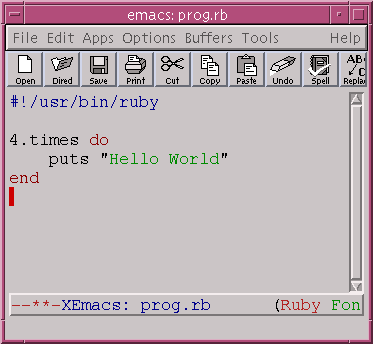Can you guess what this piece of code does? Save the file as loops.rb and run it.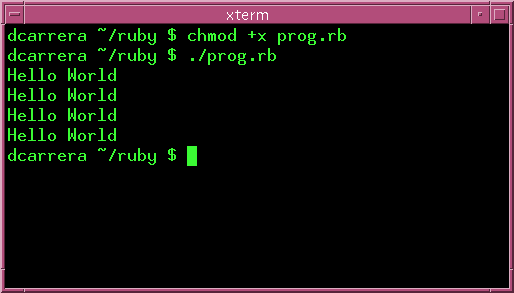As you can see, the contents of the loop have been executed 4 times. This is the most straight forward loop that Ruby offers.

## Counting

Here is another example. Now we use our knolwedge of variables to print the numbers from 1 to 5.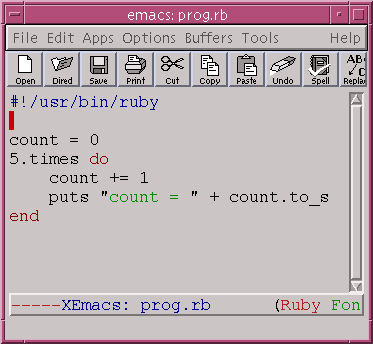Remember that the Integer#to_s method converts the integer into a string, so we can add it to the string "count = ".

When you run this you should get: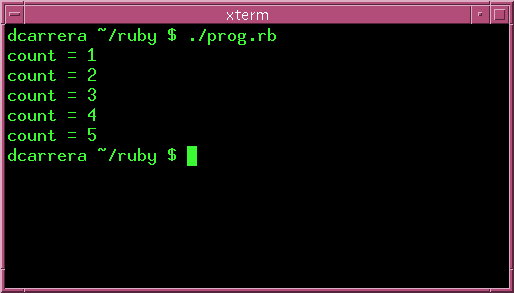## A sum of numbers

Suppose that I want to know the sum of all the numbers from 1 to 11. We already know how to get all the numbers from 1 to 11. All we really need to do is add them together: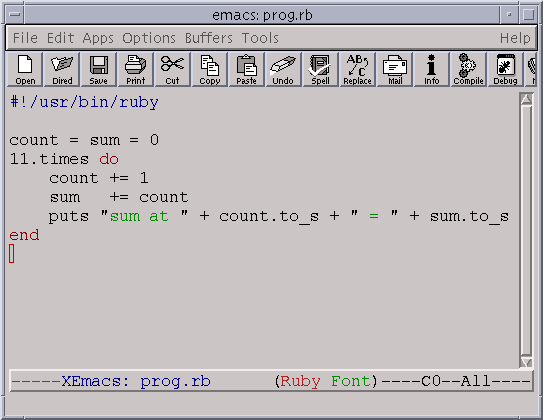You should get something like this: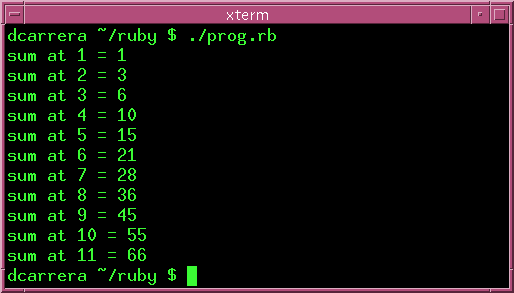## Multi-line statements.

That puts statement in the last example was getting somewhat long. What happens if you want to type a very long line?

You can make lines "wrap around" by putting a backslash - \ - at the very end of the line. Look at this example in irb.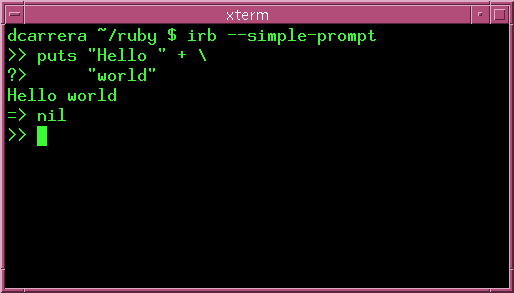That '=> nil' simply means that puts returns nothing. In other words, if you typed:

```variable = puts "hello"
```

Then variable would end up with nothing. And Ruby's object for "nothing" is nil.

What you should be paying attention to is the fact that we spread out the puts over two lines. Let's use what we just learned to rewrite that line in our program: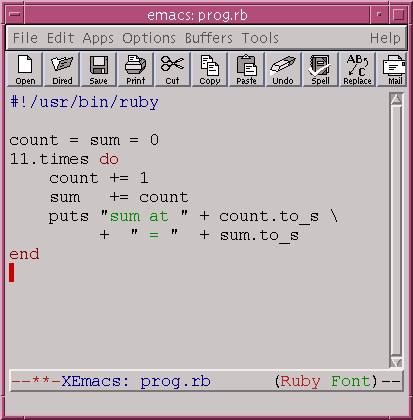Indeed, you can use this to print as much as you like: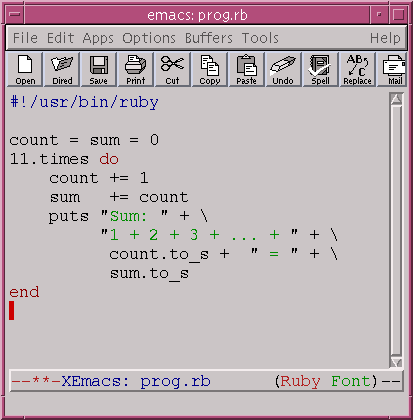Notice. You don't have to make the program line up like that. I did it because I think it looks better. When you run this program you will see: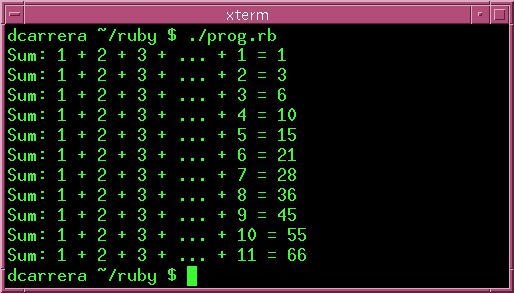Warning: The backslash '\' must be the last character in the line. If you put so much as a space after it, you will get an error.

## More examples

Let's try counting backwards.

Just to illustrate a couple of points. Here are some examples.

### Counting backwards

Type in this program: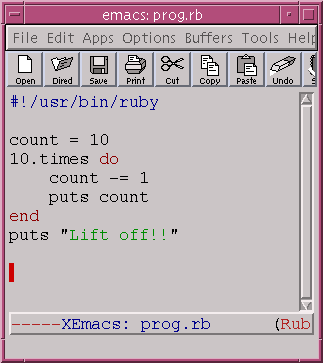This produces: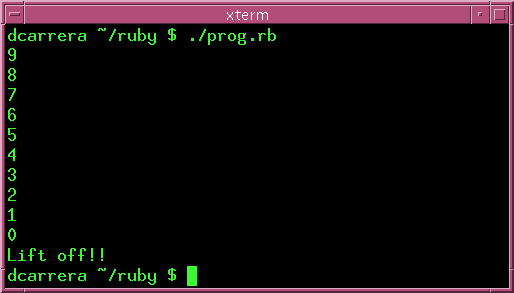### Counting a variable number of times

These loops also work with variables.

In this example, we compute the factorial of a number. The factorial of a number n is the product:

```1 x 2 x 3 x ... x n
```

The symbol for this is n!. In this example we compute.

```6! = 6 x 5 x 4 x 3 x 2 x 1 = 720
```

Type and run this program: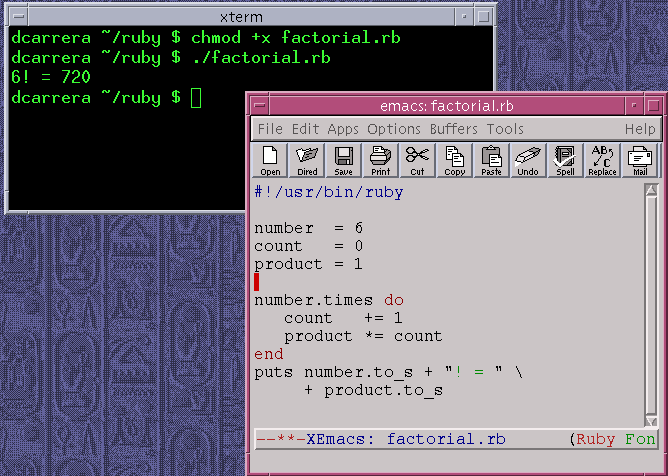## Exercises

1. What is the sum of all the integers from 1 to 1000?

2. What is the sum of all the integers from 10 to 100?

3. Print out all the lyrics to "99 bottles of beer on the wall".

4. Here is a more difficult problem.

There is a song that goes like this:

On the first day of Christmas, my true love sent to me a partridge in a pear tree.
On the second day of Christmas, my true love sent to me two turtle doves and a partridge in a pear tree.
...
If this goes on for the 12 days of Christmas. How many presents will your true love send you over Christmas?
(Hint: You will need a loop inside another).